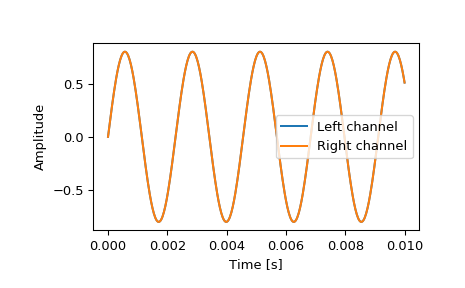scipy.io.wavfile.read(filename, mmap=False)[source]

Open a WAV file

Return the sample rate (in samples/sec) and data from a WAV file.

Parameters
filenamestring or open file handle

Input wav file.

mmapbool, optional

Whether to read data as memory-mapped. Only to be used on real files (Default: False).

New in version 0.12.0.

Returns
rateint

Sample rate of wav file.

datanumpy array

Data read from wav file. Data-type is determined from the file; see Notes.

Notes

This function cannot read wav files with 24-bit data.

Common data types: 

WAV format

Min

Max

NumPy dtype

32-bit floating-point

-1.0

+1.0

float32

32-bit PCM

-2147483648

+2147483647

int32

16-bit PCM

-32768

+32767

int16

8-bit PCM

0

255

uint8

Note that 8-bit PCM is unsigned.

References

1

IBM Corporation and Microsoft Corporation, “Multimedia Programming Interface and Data Specifications 1.0”, section “Data Format of the Samples”, August 1991 http://www.tactilemedia.com/info/MCI_Control_Info.html

Examples

>>> from os.path import dirname, join as pjoin
>>> import scipy.io as sio


Get the filename for an example .wav file from the tests/data directory.

>>> data_dir = pjoin(dirname(sio.__file__), 'tests', 'data')
>>> wav_fname = pjoin(data_dir, 'test-44100Hz-2ch-32bit-float-be.wav')


>>> samplerate, data = sio.wavfile.read(wav_fname)
>>> print(f"number of channels = {data.shape}")
number of channels = 2
>>> length = data.shape / samplerate
>>> print(f"length = {length}s")
length = 0.01s


Plot the waveform.

>>> import matplotlib.pyplot as plt
>>> import numpy as np
>>> time = np.linspace(0., length, data.shape)
>>> plt.plot(time, data[:, 0], label="Left channel")
>>> plt.plot(time, data[:, 1], label="Right channel")
>>> plt.legend()
>>> plt.xlabel("Time [s]")
>>> plt.ylabel("Amplitude")
>>> plt.show()#### Previous topic

scipy.io.hb_write

#### Next topic

scipy.io.wavfile.write